# IEEE floating-point arithmetic¶

This chapter describes functions for examining the representation of floating point numbers and controlling the floating point environment of your program. The functions described in this chapter are declared in the header file cml/ieee.h.

## Representation of floating point numbers¶

The IEEE Standard for Binary Floating-Point Arithmetic defines binary formats for single and double precision numbers. Each number is composed of three parts: a sign bit (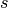), an exponent (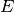) and a fraction (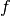). The numerical value of the combination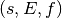is given by the following formula,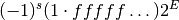The sign bit is either zero or one. The exponent ranges from a minimum value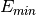to a maximum value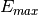depending on the precision. The exponent is converted to an unsigned number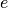, known as the biased exponent, for storage by adding a bias parameter,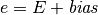The sequence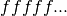represents the digits of the binary fraction. The binary digits are stored in normalized form, by adjusting the exponent to give a leading digit of. Since the leading digit is always 1 for normalized numbers it is assumed implicitly and does not have to be stored. Numbers smaller than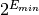are be stored in denormalized form with a leading zero,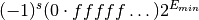This allows gradual underflow down to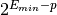for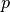bits of precision. A zero is encoded with the special exponent of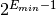and infinities with the exponent of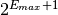.

The format for single precision numbers uses 32 bits divided in the following way:

seeeeeeeefffffffffffffffffffffff

s = sign bit, 1 bit
e = exponent, 8 bits  (E_min=-126, E_max=127, bias=127)
f = fraction, 23 bits


The format for double precision numbers uses 64 bits divided in the following way:

seeeeeeeeeeeffffffffffffffffffffffffffffffffffffffffffffffffffff

s = sign bit, 1 bit
e = exponent, 11 bits  (E_min=-1022, E_max=1023, bias=1023)
f = fraction, 52 bits


It is often useful to be able to investigate the behavior of a calculation at the bit-level and the library provides functions for printing the IEEE representations in a human-readable form.

void cml_ieee754_fprintf_float(FILE * stream, const float * x)
void cml_ieee754_fprintf_double(FILE * stream, const double * x)

These functions output a formatted version of the IEEE floating-point number pointed to by x to the stream stream. A pointer is used to pass the number indirectly, to avoid any undesired promotion from float to double. The output takes one of the following forms,

NaN

the Not-a-Number symbol

Inf, -Inf

positive or negative infinity

1.fffff...*2^E, -1.fffff...*2^E

a normalized floating point number

0.fffff...*2^E, -0.fffff...*2^E

a denormalized floating point number

0, -0

positive or negative zero

The output can be used directly in GNU Emacs Calc mode by preceding it with 2# to indicate binary.

void cml_ieee754_printf_float(const float * x)
void cml_ieee754_printf_double(const double * x)

These functions output a formatted version of the IEEE floating-point number pointed to by x to the stream stdout.

The following program demonstrates the use of the functions by printing the single and double precision representations of the fraction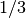. For comparison the representation of the value promoted from single to double precision is also printed.

#include <stdio.h>
#include <cml.h>

int
main(void)
{
float f = 1.0/3.0;
double d = 1.0/3.0;

double fd = f; /* promote from float to double */

printf(" f = ");
cml_ieee754_printf_float(&f);
printf("\n");

printf("fd = ");
cml_ieee754_printf_double(&fd);
printf("\n");

printf(" d = ");
cml_ieee754_printf_double(&d);
printf("\n");

return 0;
}


The binary representation ofis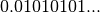. The output below shows that the IEEE format normalizes this fraction to give a leading digit of 1:

 f = 1.01010101010101010101011*2^-2
fd = 1.0101010101010101010101100000000000000000000000000000*2^-2
d = 1.0101010101010101010101010101010101010101010101010101*2^-2


The output also shows that a single-precision number is promoted to double-precision by adding zeros in the binary representation.